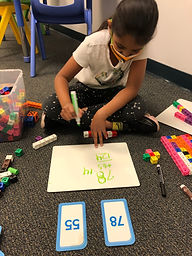Ms. Hiral

Target 1​

Lesson Type:

Continuation

Number Operation

:

Computation

Add within 1,000 (regrouping limited to only one place-value).

1:

Understand that when adding a multi-digit number, each place-value is being added individually to create the new sum (ones are added to ones, tens are added to tens, etc.)

2:

Understand that when adding a multi-digit number, to solve start in the lowest place-value and go left.

3:

Understand how to regroup based on place-value understandings (i.e., ten ones can be composed into one ten – ten tens can be composed into one hundred).

4:

Understand that addition equations can be written both vertically and horizontally.

5:

Understand that the same process is utilized to add three or more whole numbers that is used to add only two numbers.

1st

Vocabulary:

Activities:

Students practiced writing double and triple digit addition equations vertically and horizontally. Students practiced double digit addition and regrouping using blocks. Students practiced regrouping based on place-value (ten ones turns into one ten) using base 10 and ones blocks. Students practiced solving double digit addition equations using the numbers they were given though number cards.Home Exploration

Guiding Questions:Absent Students:

Target 2

:

Vocabulary:

Activities:Home Exploration

Guiding Questions:Target 3

:

Vocabulary:

Activities:Home Exploration